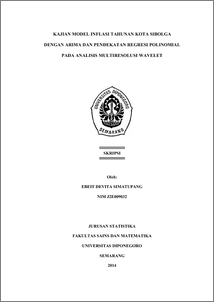# KAJIAN MODEL INFLASI TAHUNAN KOTA SIBOLGA DENGAN ARIMA DAN PENDEKATAN REGRESI POLINOMIAL PADA ANALISIS MULTIRESOLUSI WAVELET

SIMATUPANG, EBEIT DEVITA (2014) KAJIAN MODEL INFLASI TAHUNAN KOTA SIBOLGA DENGAN ARIMA DAN PENDEKATAN REGRESI POLINOMIAL PADA ANALISIS MULTIRESOLUSI WAVELET. Undergraduate thesis, FSM Undip.Preview
PDF
1052Kb

## Abstract

Inflation rate is one of the fundamental economic indicators of a country. Therefore, prediction of inflation rate become important thing in taking monetary to maintain economy stability. In studying inflation model, commonly used method of parametric ARIMA Box-Jenkins which requires data is stationer and residual is white noise. However, data inflation which is fluctuates often does not meet parametric assumptions. In this study, it is proposed to use wavelet Multiresolution Analysis (MRA) as alternative method. The transformation from wavelet capable in representing time and frequencies simultaneously so that it can be used to analyze nonstasioner data. One of wavelet transformation form is discrete wavelet transformation (DWT) which expresses sized data N as 2^J for positive integer j. DWT analyses supported by MRA that divides data X become detail component (D_j) and smoothing component (S_j) to gain of estimating result. The best of MRA estimation will be approached by polynomial regression. The model of regression is formed by summing influence each variable predictor which raised increasingly to k-degress. By using yoy inflation data of Sibolga City in July 2008-October 2013 period, obtain the best parametric model ARIMA (0,1,) with MSE=1,15411 and the best model of polynomial regression approached 13-degress at MRA that use la18 filter in resolution level j=1 which has MSE=1,238816. Both models are used to forecast yoy inflation of Sibolga City in 2014. Keywords: yoy Inflation of Sibolga City, ARIMA, Multiresolution Analysis (MRA), Polynomial Regression.

Item Type: Thesis (Undergraduate) H Social Sciences > HA Statistics Faculty of Science and Mathematics > Department of Statistics 43456 Mr Hasbi Yasin 12 Aug 2014 11:23 12 Aug 2014 12:21

Repository Staff Only: item control page